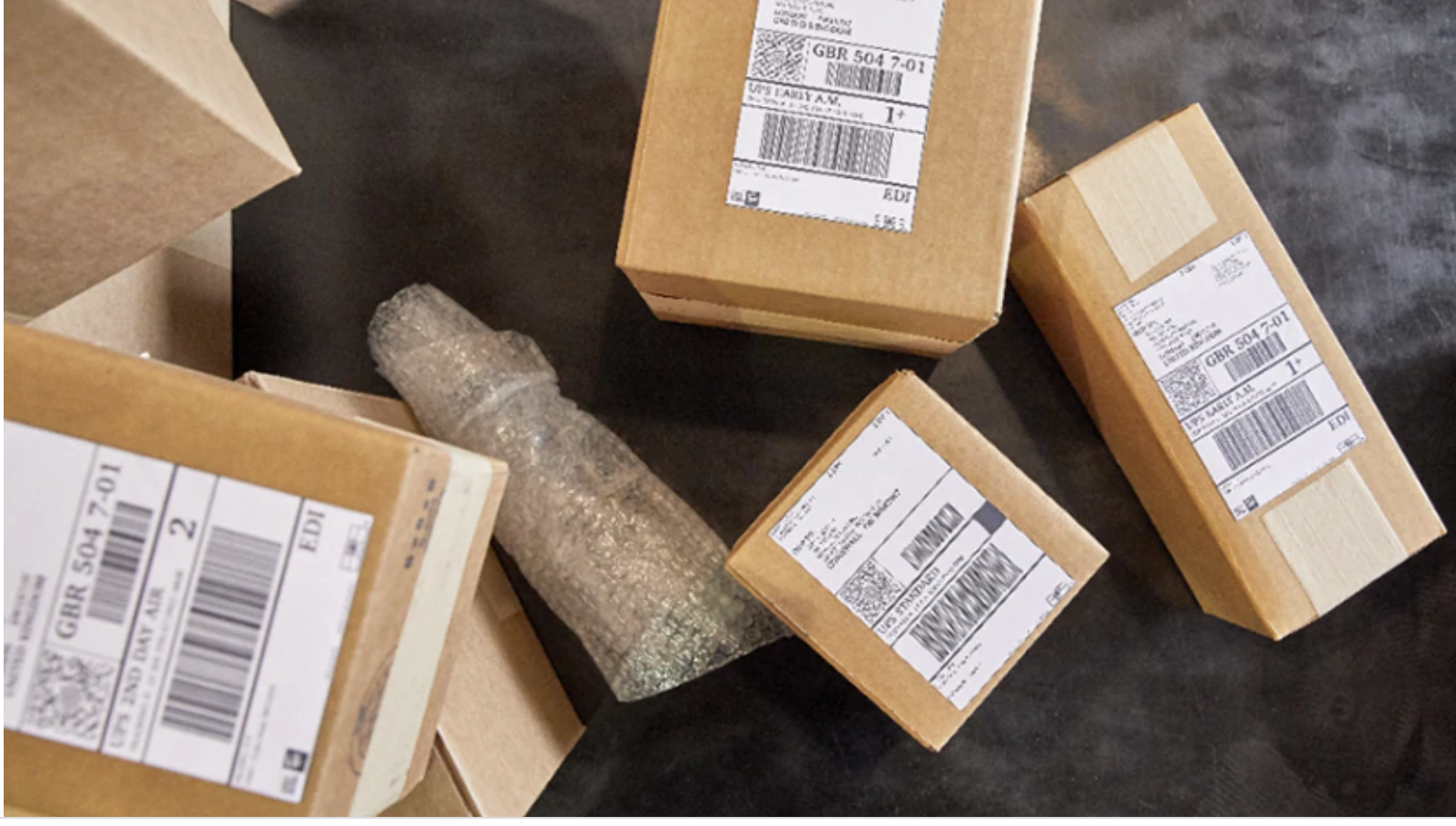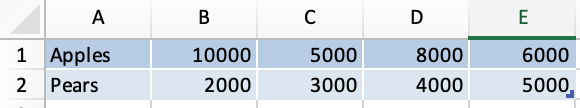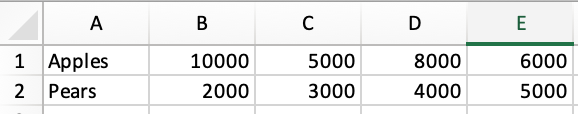Rewriting an Existing Python ProgramRecently, I have been involved in re-writing several Python programs. I’ve learned a lot and there are some thoughts I’d like to share with the data science community.

Signs that re-writing an existing package is a great idea

You can make it faster/simpler/more modern

For example, in terms of writing data to file, for convenience purpose, using pandas.DataFrame.to_excel might be simpler than using xlsxwriter (if you don’t want to control the font and width or color of the columns). Take a look of below code snippet.

data = [
['Apples', 10000, 5000, 8000, 6000],
['Pears', 2000, 3000, 4000, 5000],
]

Option 1 (at least 4 lines of code, with fancy style formatting it can be more lengthy): use xlsxwriter

# example 1
import xlsxwriter
workbook =xlsxwriter.Workbook('table_using_xlsxwriter.xlsx')
workbook.close()Option 2 (can be as simple as 2 lines):use pandas

# example 2
import pandas as pd
df = pd.DataFrame(data)The two different implementations have exactly the same output, but the latter one is clearly simpler. Another example can be found here.

There is room for improvement, re-writing makes it easier to read

You have a pure function calc_var4 that looks like below, and the goal is to make a new column (“var4”) within a dataframe df using the three columns “var1”, “var2”, and “var3”.

# example 3
def calc_var4(var1, var2, var3):
"""a simple math calculation"""
var4 = 4*var1 +5 * var2 -var3
return var4

The original code might look like this:

# example 4
def calc_var4_in_df(df):
"""compute var4 column using columns in df"""
var4_lst = []
for row in range(len(df)):
row_var4 = calc_var4(df.iloc[row]['var1'],df.iloc[row]['var2'], df.iloc[row]['var3'])
var4_lst.append(row_var4)
return var4_lst

# add var4 column to df
df['var4'] =calc_var4_in_df(df)

The solution works perfectly fine. But is it the best solution? Numpy(Pandas) Vectorized Method is a way faster implentation that doesn’t even require the level of overthinking of the original code.

#example 5
df['var4'] = 4*df['var1'] + 5 * df['var2'] - df['var3']

Ta-da, now you can easily read how var4 is related to var1, var2, and var3 that doesn’t need calc_var4 or calc_var4_in_df.

In a fine level, you want to really understand how the code works

What does the variable df look like? Does df have redundant columns? Is corrected_df_average a vector or a scaler? What intermediate values were generated and how were they used in the downstream analysis? Even though you might understand the big picture, you might still have tons of questions about how it actually works. Playing around the code would give you a better understanding.

You want to propose potential upgrade or extension to the code base

This part comes naturally once you understand the steps and execution of each function/class/module, you’ll have a good idea of where to insert additional pieces to enhance the program.

What to consider when re-writing an existing package

Knowing your goal, so that you’ll know how much time you’d want to invest

Do you want to increase the computational speed or just clean up redundant code to have a better documentation? Knowing your goal gives you an estimate on how much time you’d want to spend in the re-writing process. After all, the code is already working no matter how slow or not pretty it is.

One great example of how re-writing existing code could significantly increase the computational speed is jax-unirep, made by my friends Eric Ma and Arkadij Kummer.jax-unirep speeds up the original unirep developed in George Church’s lab for protein sequence representation. By looking at one and ten random protein sequences of length ten (Figure 5 in paper), jax-unirep paper showed a drastic improvement of speed.

In terms of making the code look nicer, my friend Alok Saldanha recommended a book called Professor Frisby’s Mostly Adequate Guide to Functional Programming. This book uses examples in Java and promotes the idea of writing pure functions, which eliminates a function’s side effects and its affect on mutable state. For example, in “example 4” above, one can put df['var4'] =calc_var4_in_df(df) inside of calc_var4_in_df and return mutated df instead, but it is not recommended since an external dataframe df will be mutated as a result.

Knowing what to test for and test frequently

Unless we identify bugs, we hope to see the outputs of re-writing to be the same as the original version. Testing is an important step to ensure everything works the same, but it can be a pain given the complexity of the code.

• Write pure and testable functions

Following the advice of Chapter 03: Pure Happiness with Pure Functions of the book by Professor Frisby, we would want a function to be cacheable, self-contained and self-documenting, testable and reasonable.

• If only certain functions were re-written, first test if the output from the new function is the same as the old one. For example, using the same input df, is the df returned by exmaple 4 same as example 5?

• Have a simple and representative input for end-to-end testing

Many times things aren’t that simple. You might encounter the original code to be like this

# example 6
class SomeMathWork:
def __init__(self, a, b):
self.a = a
self.b = b

def func_x(self):
return self.a *4 +self.b*5

def func_y(self):
c = self.func_x()
d = 6*c +1
return d

If it turns out that you’d want to re-implement func_x, you probably will need to test if func_y returns the same.

#example 7
old_mathwork = SomeMathWork(1,2)
old_output_x = old_mathwork.func_x()
old_output_y = old_mathwork.func_y()

new_mathwork = SomeMathWork(1,2)
new_output_x = new_mathwork.func_x()
new_output_y = new_mathwork.func_y()

assert(old_output_x == new_output_x)
assert(old_output_y == new_output_y)

You can use other fancy framework such as pytest to make automated tests.